# Question 2 Performance of Compilers [25 pts.) In this question, we are given two different compilers...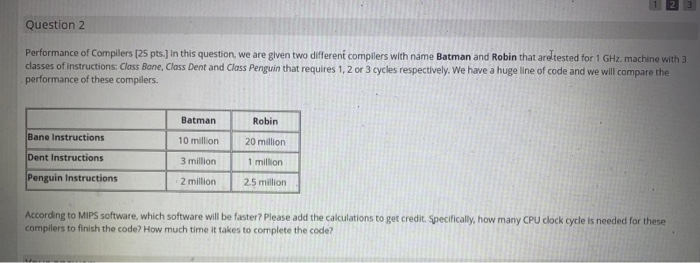Question 2 Performance of Compilers [25 pts.) In this question, we are given two different compilers with name Batman and Robin that are tested for 1 GHz. machine with 3 classes of instructions: Class Bane, Class Dent and Class Penguin that requires 1, 2 or 3 cycles respectively. We have a huge line of code and we will compare the performance of these compilers. Batman Robin Bane Instructions 10 million 20 million Dent Instructions 3 million 1 million Penguin Instructions 2 million 2.5 million According to MIPS software, which software will be faster? Please add the calculations to get credit Specifically, how many CPU clock cycle is needed for these compilers to finish the code? How much time it takes to complete the code?

For Batman
Total clock = 10*1 + 3*2 + 2*3 = 22 millions
Total instruction = 15 millons
average clock per Instruction = (22*10^6)/(15*10^6) = 22/15
MIPS = 10^9/(22/15) = (15000/22)*10^6 = 681.81 MIPS
execution time = 22*10^6/(10^9) = 0.022 seconds

For Robin
Total clock = 20*1 + 1*2 + 2.5*3 = 29.5 millions
Total instruction = 23.5 millons
average clock per Instruction = (29.5*10^6)/(23.5*10^6) = 29.5/23.5
MIPS = 10^9/(29.5/23.5) = 796.61 MIPS
execution time = 29.5*10^6/(10^9) = 0.0295 seconds

According to MIPS , software Robin is faster (796.61 > 681.81)

#### Earn Coin

Coins can be redeemed for fabulous gifts.

Similar Homework Help Questions
• ### Performance of Compilers [25 pts.] In this question, we are given two different compilers with name...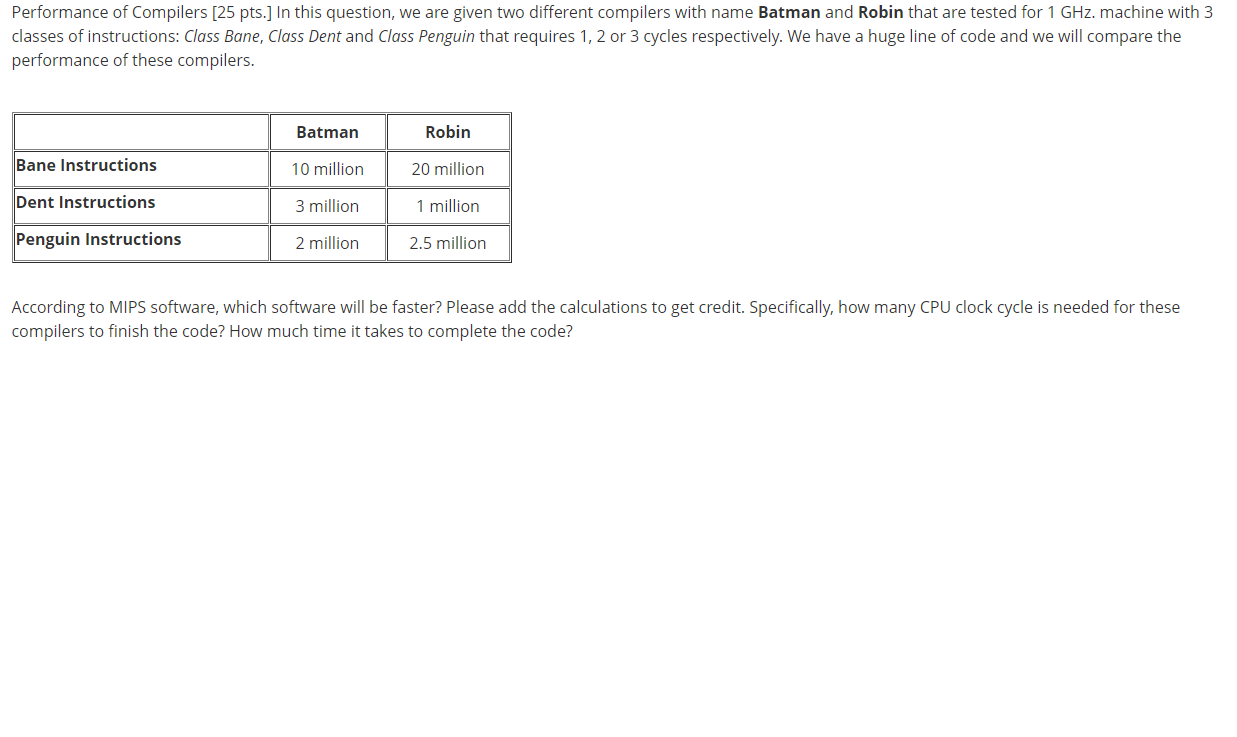Performance of Compilers [25 pts.] In this question, we are given two different compilers with name Batman and Robin that are tested for 1 GHz. machine with 3 classes of instructions: Class Bane, Class Dent and Class Penguin that requires 1, 2 or 3 cycles respectively. We have a huge line of code and we will compare the performance of these compilers. Batman Robin Bane Instructions 10 million 20 million Dent Instructions 3 million 1 million Penguin Instructions 2 million...

• ### I need help with the following Computer Architecture question: Consider two different implementations, M1 and M2,...

I need help with the following Computer Architecture question: Consider two different implementations, M1 and M2, of the same instruction set. There are three classes of instructions (A, B, and C) in the instruction set. M1 has a clock rate of 90 MHz and M2 has a clock rate of 80 MHz. The average number of cycles for each instruction class and their frequencies (for a typical program) are as follows: Instruction Class Machine M1 – Cycles/Instruction Class Machine M2...

• ### We are comparing the performance of two different microprocessors, M1 and M2. Assume a program P...

We are comparing the performance of two different microprocessors, M1 and M2. Assume a program P will be compiled into M2 instructions counting 20% more than M1 instructions. M1 has a 2 GHz clock rate while M2 has a 3 GHz clock rate. Instructions on these two machines can be classified into 3 classes as shown in the following table. Which machine has better MIPS? Which machine has better performance? By how much? Justify your answers. Instruction Class Cycles needed...

• ### (e) Suppose we measure the code for the same program from two different compilers and obtain the following data. Assume clock rate is 3GHz, which code sequence will execute faster according to ex...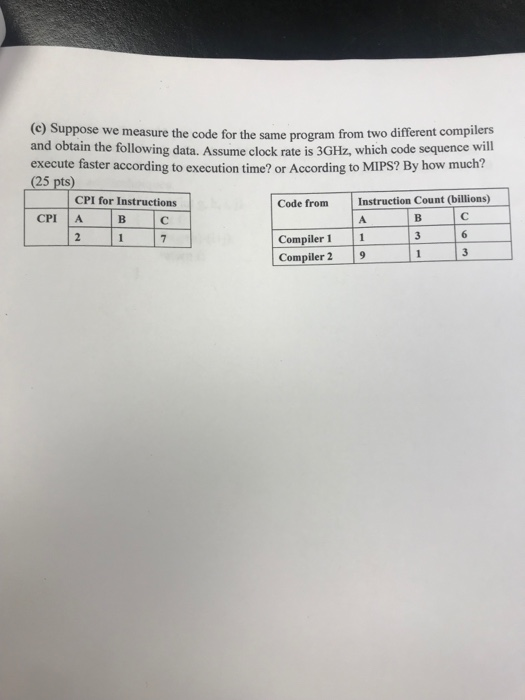(e) Suppose we measure the code for the same program from two different compilers and obtain the following data. Assume clock rate is 3GHz, which code sequence will execute faster according to execution time? or According to MIPS? By how much? (25 pts CPI for Instructions Code from Instruction Count (billions) CPI Compiler 1 Compiler 2 9 1 3 (e) Suppose we measure the code for the same program from two different compilers and obtain the following data. Assume clock...

• ### Design and implement a C Language program that measures the performance of given processors. There are...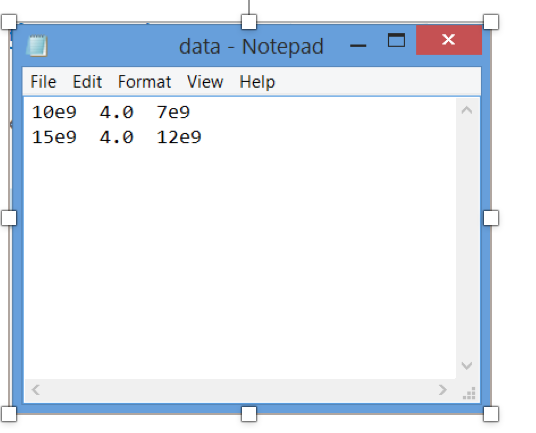Design and implement a C Language program that measures the performance of given processors. There are several metrics that measure the performance of a processor. We will be using the following 3 measures: 1.CPI (clock cycles per instruction) = #clock cycles /#instructions 2.CPU execution time = #instructions x CPI x clock cycle time . cylce time = 1/CPU clock rate in hertz units 3.MIPS (mega instructions per second)= #instrucrions/ CPU X 1000000 Typically, processors’ performance is measured using a wide...

• ### Problem 3. (25 pts.) Compilers can have a profound impact on the performance of an application....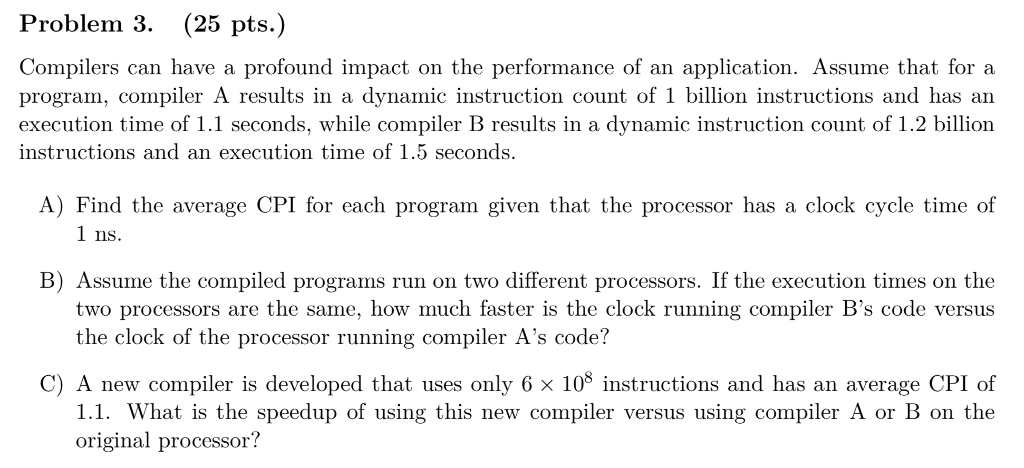Problem 3. (25 pts.) Compilers can have a profound impact on the performance of an application. Assume that for a program, compiler A results in a dynamic instruction count of 1 billion instructions and has an execution time of 1.1 seconds, while compiler B results in a dynamic instruction count of 1.2 billion instructions and an execution time of 1.5 seconds. A) Find the average CPI for each program given that the processor has a clock cycle time of 1...

• ### In the class, we have shown how to maximize performance on our pipelined datapath with forwarding...

In the class, we have shown how to maximize performance on our pipelined datapath with forwarding and stalls on a use following a load. Rewrite the following code to minimize performance on this datapath – that is, reorder the instructions so that this sequence takes the most clock cycles to execute while still obtaining the same result: lw \$3, 0(\$5)  lw \$4, 4(\$5)  add \$7, \$7, \$3 add \$8, \$8, \$4 add \$10, \$7, \$8 sw \$6, 0(\$5)  beq \$10,...

• ### second attempt. need asap please 2-4 sentences summarizing the article 4 interesting quotes from the article...

second attempt. need asap please 2-4 sentences summarizing the article 4 interesting quotes from the article and 4 points explaining each quote In the first few years of the new millennium, at the height of the boom in the offshore call-center business, Tata Consultancy Services, the Indian technology-services giant, made the counterintuitive decision to divest its call-center operations. Why? Because although outsourced call centers were a fast-growing piece of its current business, TCS’s leadership had come to believe that they...

• ### How can we assess whether a project is a success or a failure? This case presents...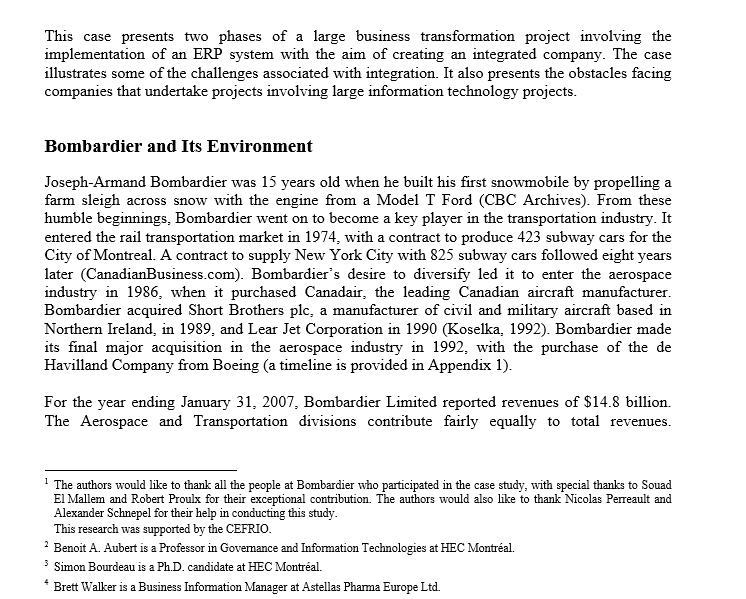How can we assess whether a project is a success or a failure? This case presents two phases of a large business transformation project involving the implementation of an ERP system with the aim of creating an integrated company. The case illustrates some of the challenges associated with integration. It also presents the obstacles facing companies that undertake projects involving large information technology projects. Bombardier and Its Environment Joseph-Armand Bombardier was 15 years old when he built his first snowmobile...

Free Homework Help App